LSMEANS Statement
LSMEANS fixed-effects </ options> ;

The LSMEANS statement computes least squares means (LS-means) of fixed effects. As in the GLM procedure, LS-means are predicted population margins—that is, they estimate the marginal means over a balanced population. In a sense, LS-means are to unbalanced designs as classification and subclassification arithmetic means are to balanced designs. Thematrix constructed to compute them is the same as thematrix formed in PROC GLM; however, the standard errors are adjusted for the covariance parameters in the model.

Each LS-mean is computed as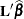, whereis the coefficient matrix associated with the least squares mean and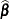is the estimate of the fixed-effects parameter vector. The approximate standard errors for the LS-mean is computed as the square root of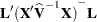.

LS-means can be computed for any effect in the MODEL statement that involves CLASS variables. You can specify multiple effects in one LSMEANS statement or in multiple LSMEANS statements, and all LSMEANS statements must appear after the MODEL statement. As in the ESTIMATE statement, thematrix is tested for estimability, and if this test fails, PROC HPMIXED displays "Non-est" for the LS-means entries.

Assuming the LS-mean is estimable, PROC HPMIXED constructs an approximate t test to test the null hypothesis that the associated population quantity equals zero. By default, the denominator degrees of freedom for this test are the same as those displayed for the effect in the "Type III Tests of Fixed Effects" table (see the section TEST Statement).

You can specify the following options in the LSMEANS statement after a slash (/).

ALPHA=number

requests that a t-type confidence interval be constructed for each of the LS-means with confidence level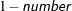. The value of number must be between 0 and 1; the default is 0.05.

CL

requests that t-type confidence limits be constructed for each of the LS-means. If DDFM=NONE, then PROC HPMIXED uses infinite degrees of freedom for this test, essentially computing a z interval. The confidence level is 0.95 by default; this can be changed with the ALPHA= option.

CORR

displays the estimated correlation matrix of the least squares means as part of the "Least Squares Means" table.

COV

displays the estimated covariance matrix of the least squares means as part of the "Least Squares Means" table.

DF=number

specifies the degrees of freedom for the t test and confidence limits. The default is the denominator degrees of freedom taken from the "Type III Tests of Fixed Effects" table corresponding to the LS-means effect. For these DDFM= methods, degrees of freedom are determined separately for each test; see the DDFM= option for more information.

DIFF<=difftype>
PDIFF<=difftype>

requests that differences of the LS-means be displayed. You can specify the following values for the optional difftype.

DIFF=ALL

requests all pairwise differences; it is the default.

DIFF=ANOM

requests differences between each LS-mean and the average LS-mean, as in the analysis of means (Ott; 1967). The average is computed as a weighted mean of the LS-means, with the weights being inversely proportional to the diagonal entries of the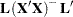matrix. When a WEIGHT statement is specified, then the preceding matrix is replaced with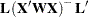where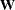is the diagonal matrix that contains the weights. If LS-means are nonestimable, this design-based weighted mean is replaced with an equally weighted mean. Note that the ANOM procedure in SAS/QC software implements both tables and graphics for the analysis of means with a variety of response types. For one-way designs and normally distributed data, the DIFF=ANOM computations are equivalent to the results of PROC ANOM.

DIFF=CONTROL

requests differences with a control; by default, the control is the first level of each of the specified LSMEANS effects. To specify which levels of the effects are the controls, list the quoted formatted values in parentheses after the CONTROL keyword. For example, if the effects A, B, and C are classification variables, each having two levels, 1 and 2, the following LSMEANS statement specifies the (1,2) level of A*B and the (2,1) level of B*C as controls:

```lsmeans A*B B*C / diff=control('1' '2' '2' '1');
```

For multiple effects, the results depend upon the order of the list, and so you should check the output to make sure that the controls are correct.

CONTROL produces two-tailed tests and confidence limits.

DIFF=CONTROLL

requests one-tailed results and tests whether the noncontrol levels are significantly smaller than the control. The upper confidence limits for the control minus the noncontrol levels are considered to be infinity and are displayed as missing.

DIFF=CONTROLU

requests one-tailed results and tests whether the noncontrol levels are significantly larger than the control. The upper confidence limits for the noncontrol levels minus the control are considered to be infinity and are displayed as missing.

The differences of the LS-means are displayed in a table titled "Differences of Least Squares Means." For ODS purposes, the table name is "Diffs."

E

requests that the matrix coefficients for all LSMEANS effects be displayed. For ODS purposes, the name of this "Matrix Coefficients" table is "Coef."

PDIFF

is the same as the DIFF option.

SINGULAR=number

tunes the estimability checking as documented for the SINGULAR= in the CONTRAST statement.

SLICE=fixed-effect
SLICE=(fixed-effects)

specifies effects by which to partition interaction LSMEANS effects. This can produce what are known as tests of simple effects (Winer; 1971). For example, suppose that A*B is significant, and you want to test the effect of A for each level of B. The appropriate LSMEANS statement is

```lsmeans A*B / slice=B;
```

This statement tests for the simple main effects of A for B, which are calculated by extracting the appropriate rows from the coefficient matrix for the A*B LS-means and by using them to form an F test.

The SLICE= option produces F tests that test the simultaneous equality of cell means at a fixed level of the slice effect (Schabenberger, Gregoire, and Kong; 2000).

The SLICE= option produces a table titled "Tests of Effect Slices." For ODS purposes, the table name is "Slices."